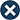Try AccountingSuite™
No credit card needed

# Economic Order Quantity (EOQ)

Economic Order Quantity (EOQ): The optimal order quantity that minimizes inventory holding costs and ordering costs.

Economic Order Quantity (EOQ) is a mathematical formula used in inventory management to determine the optimal order quantity that minimizes inventory holding costs and ordering costs. EOQ is based on the assumption that there is a tradeoff between the costs of holding inventory and the costs of ordering inventory.

The EOQ formula takes into account several variables, including the cost of ordering inventory, the cost of holding inventory, and the demand for the product. The formula calculates the optimal order quantity that minimizes the total cost of inventory management.

The primary advantages of using EOQ in inventory management include:

1. Reduced inventory holding costs: EOQ helps businesses reduce the costs of holding inventory by minimizing the amount of inventory on hand.
2. Reduced ordering costs: EOQ helps businesses reduce the costs of ordering inventory by optimizing the order quantity and frequency.
3. Improved inventory management: EOQ helps businesses optimize their inventory management processes by ensuring that inventory levels are optimal for meeting customer demand.

There are some limitations to using EOQ in inventory management, including:

1. Assumptions may not always hold: EOQ assumes that demand is constant and that costs are known and stable, which may not always be the case in real-world inventory management.
2. Limited applicability: EOQ is most effective for managing inventory of products with stable demand patterns and known costs.
3. Complex calculation: EOQ requires a relatively complex mathematical formula, which may be challenging for some businesses to implement.

Overall, EOQ is an effective tool for businesses to optimize inventory management and reduce costs. By carefully analyzing demand patterns, ordering costs, and inventory holding costs, businesses can determine the optimal order quantity that balances these factors and achieves the most efficient inventory management possible.